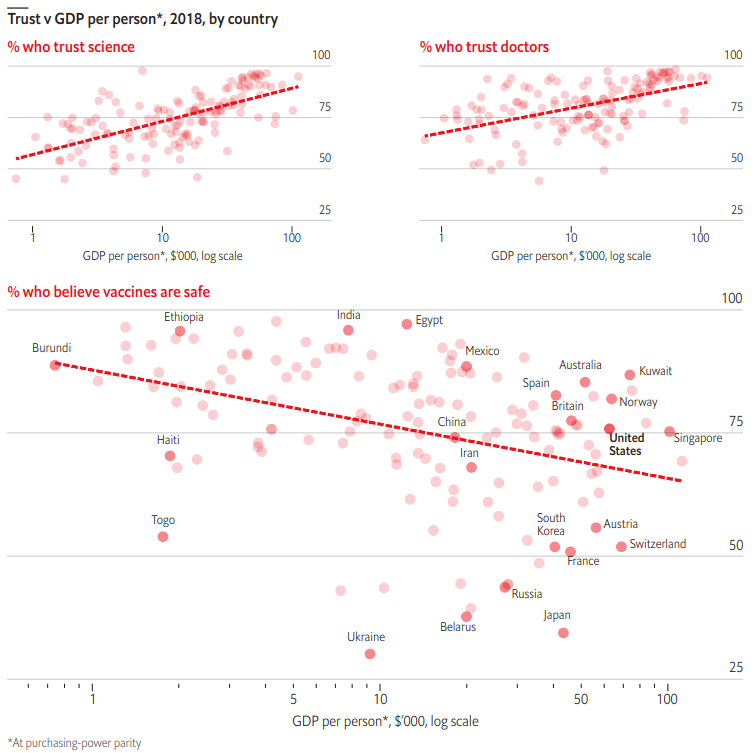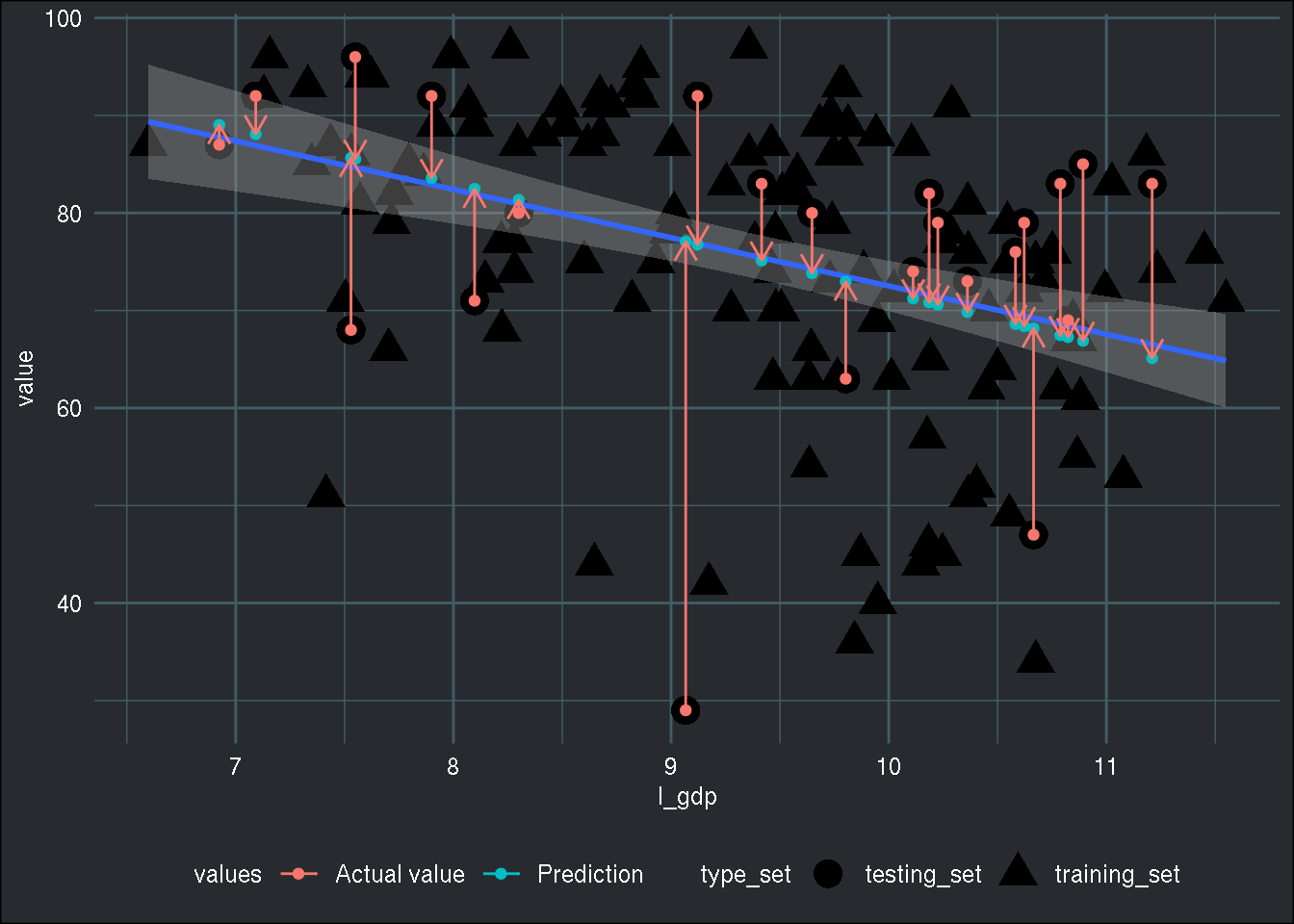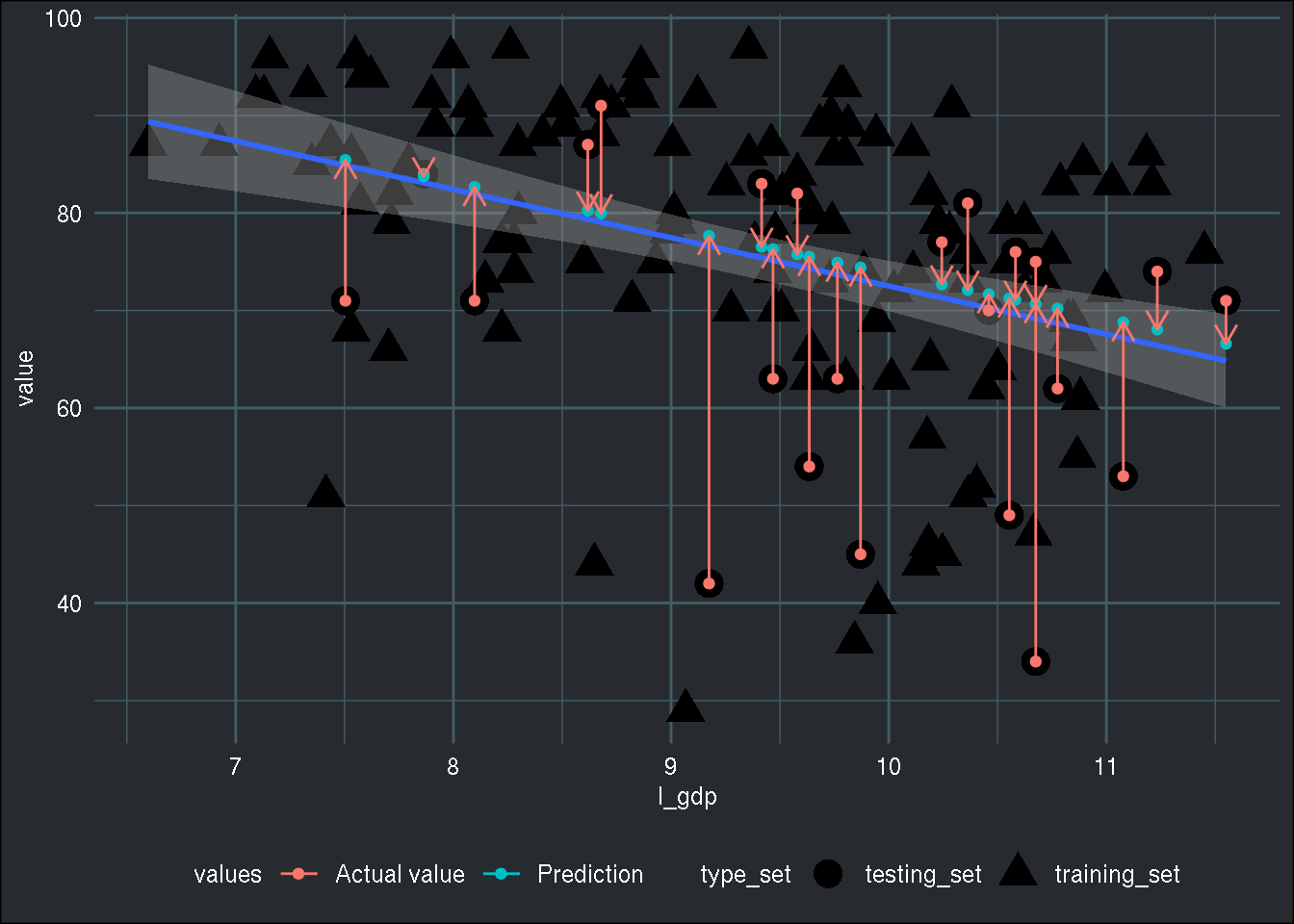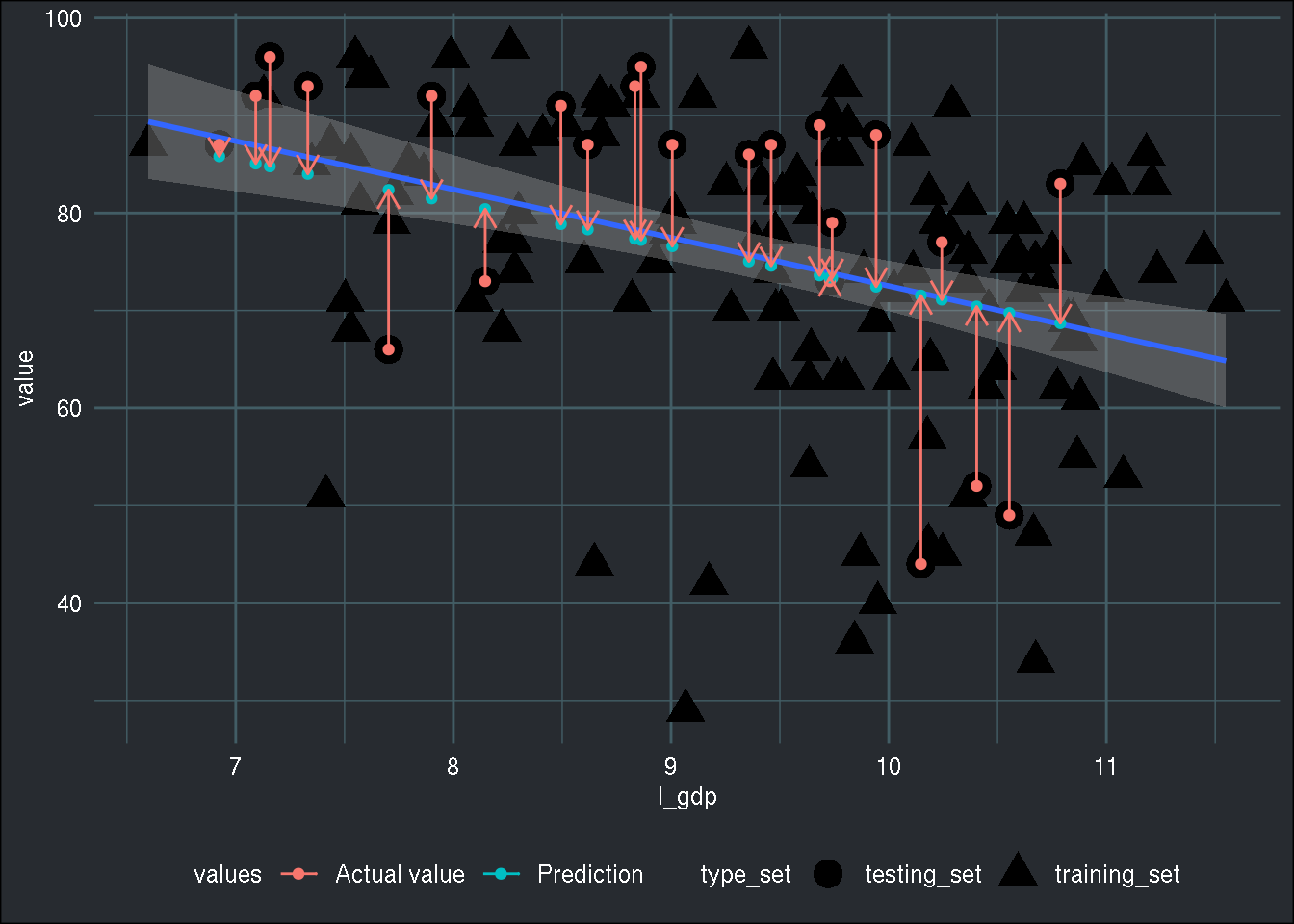Econometrics and Free Software by Bruno Rodrigues.
Check out my package that adds logging to R functions, {chronicler}.
Or read my free ebooks, to learn some R and build reproducible analytical pipelines..
You can also watch my youtube channel.
Buy me a coffee, my kids don't let me sleep.


# (Half) Lies, (half) truths and (half) statistics

R

Note: if you’re reading this and images are not showing, visit the original post on my blog. The blog post contains interactive plots which help in understanding the point I’m making.

I’ve recently come across this graph (on Twitter) from the Economist:You can read the article here (archived for posterity). There are many things wrong with this chart. First of all, the economist is fitting a linear regression to some data points, and does not provide anything else to the reader, namely the regression coefficients, their standard errors, and the R². I know already that some readers will disagree, thinking something along the lines of “But Bruno, come on, this is only to show that’s there a negative correlation between GDP per capita and trust in vaccines! The readers don’t need to be bothered with these complex concepts. This is just an illustration, and it’s good enough.”

WRONG.

Look, I’m all for good enough. That’s very likely going to be my epitaph. But sometimes, you can’t simplify things so much that they’re not only misleading, but lies. In this case here, the relationship between GDP per capita and trust in vaccines, if there is any, is probably highly nonlinear, and very difficult to pinpoint with any degree of accuracy. But before going further, let’s get the data and replicate the graph. I’ll be adding the equation of the regression line as well as the R² to the plot. I won’t comment my code, since the point of this blog post is not to teach you how to do it, but of course, you’re very welcome to reproduce the analysis.

You can download the data here, under “Full dataset for this chart”. You can also grab a csv version here.

Click to see the code
library(tidyverse)
library(ggiraph)

dataset <- dataset %>%
filter(grepl("(GDP per capita)|(Q25)", question_statistic)) %>%
mutate(response_type = ifelse(response_type == "", "GDP per capita", response_type)) %>%
filter(grepl("(National Total)|(GDP)", response_type)) %>%
mutate(response_type = str_remove(response_type, "National Total: ")) %>%
select(country_name, response = response_type, value = result_percent) %>%
mutate(gdp_per_capita = ifelse(grepl("GDP", response), value, NA)) %>%
fill(gdp_per_capita, .direction = "down") %>%
filter(!grepl("GDP", response))  %>%
mutate(gdp_per_capita = as.numeric(gdp_per_capita),
value = as.numeric(value),
l_gdp = log(gdp_per_capita))
plot_data <- dataset %>%
mutate(agree = ifelse(grepl(" agree$", response), "safe", "not_safe")) %>% group_by(country_name, l_gdp, agree) %>% summarise(value = sum(value)) %>% filter(agree == "safe") ## summarise() regrouping output by 'country_name', 'l_gdp' (override with .groups argument) lin_mod <- lm(value ~ l_gdp, data = plot_data) lin_mod_coefs <- coefficients(lin_mod) lin_mod_se <- sqrt(diag(vcov(lin_mod))) regression_line_result <- paste0("value = ", round(lin_mod_coefs, 2), "[", round(lin_mod_se, 2), "]", round(lin_mod_coefs, 2), "[", round(lin_mod_se, 2), "]", "*l_gdp", ",\n R2 = ", round(summary(lin_mod)$r.squared, 2))

my_plot <- plot_data %>%
ggplot(aes(y = value, x = l_gdp)) +
geom_point_interactive(aes(tooltip = country_name), colour = "orange") +
#geom_point() +
geom_smooth(method = "lm", se = FALSE) +
#ggrepel::geom_label_repel(aes(label = country_name)) +
geom_text(y = 35, x = 8,
label = regression_line_result,
colour = "white",
size = 3) +
brotools::theme_blog()

If you look at the code above, you’ll see that I’m doing a bunch of stuff to reproduce the graph. Let’s take a look at it (you can mouse over the points to see the country names over the labels):

girafe(ggobj = my_plot, width_svg = 8)
## geom_smooth() using formula 'y ~ x'

So what’s actually going on? value is the percentage of people, in a country, that believe vaccines are safe. l_gdp is the logarithm of GDP per capita in that same country. Looking at this, many people will conclude that the richer the country, the less people trust vaccines. This is the story the Economist is telling its readers. This is a simple explanation, and it’s backed by numbers and stats, so it must be correct. Right?

WRONG.

Let’s take a look at the regression equation (standard errors in square brackets):

$\text{value} = 122.04[9.3] - 4.95[0.98] * \text{l_gdp}$

Both coefficients are significant at the usual 5% level (the intercept is interesting though, as it implies a value greater than 100 for very low levels of log of GDP). This gives comfort to the person believing the basic story.

But take a look at the R². It’s 0.15. That means that the linear regression will be able to predict up to 15% of the variance in the dependent variable using the log of GDP per capita as a predictor. That already should sound all sorts of alarms in your head (if that scatter plot that looks almost like random noise didn’t already). However, I’m not done yet.

What if you wanted to do something a little bit more elaborate? For instance, let’s say that you’d like to see if infant mortality plays a role? After all, you could argue that in very poor countries, where people seem to trust vaccines very much, infant mortality is very high. Vaccinating your kid seems like a no-brainer when the alternative is almost certain death from any of the many diseases afflicting children (don’t get me wrong here, vaccinating children against deadly diseases is a no-brainer anywhere on the planet). Maybe people in wealthier countries don’t ascribe low infant mortality to vaccines, but to other things such as access to clean water, good infrastructure etc, and thus tend to downplay the role of vaccines. Who knows. But let’s dig deeper and get some more data.

For this I’m using another data set that gives the infant mortality rate in 2018 for most of the countries from the original analysis. I got that data from the Worldbank, and you can easily download the csv from here.

Below, I’m downloading the data and joining that to my original dataset. Then I’m computing a rank based on the median infant mortality rate. Countries that have an infant mortality rate below the median are classified as “low infant mortality rate” countries and countries that have an infant mortality rate above the median infant mortality rate are classified as “high infant mortality rate” countries. I then redo the same plot as before, but I’m computing one regression line per group of countries.

Click to see the code
infant_mortality_rate <- data.table::fread("https://gist.githubusercontent.com/b-rodrigues/33f64ce6910e6ec4df9d586eacf335c2/raw/01df8977edd3924a3687f783e7e5a134d5f3fd87/infant_mortality_rate_2018.csv") %>%
janitor::clean_names() %>%
select(country_name, imr = x2018_yr2018)

plot_data_simpson <- plot_data %>%
ungroup() %>%
left_join(infant_mortality_rate) %>%
mutate(imr = as.numeric(imr)) %>%
filter(!is.na(imr)) %>%
mutate(rank = ntile(imr, n = 2))  %>%
mutate(rank = ifelse(rank == 2,
"High infant mortality rate",
"Low infant mortality rate"))
## Joining, by = "country_name"
my_plot <- plot_data_simpson %>%
ggplot(aes(y = value, x = l_gdp)) +
geom_point_interactive(aes(tooltip = country_name, colour = rank)) +
geom_smooth(aes(group = rank), method = "lm") +
brotools::theme_blog()
girafe(ggobj = my_plot, width_svg = 8)
## geom_smooth() using formula 'y ~ x'

All of a sudden, the relationship turns positive for high income countries. This is the famous Simpson’s paradox in action. If you don’t know about Simpson’s paradox, you can read about it here.

Now what? Should we stop here? No.

Let’s not even consider Simpson’s paradox. Even though the authors never claim to have found any causal mechanism (and the Economist made no such claim, even though they tried hard to find some after the fact explanation to justify their findings), authors of such studies do very often imply that their simple analysis has at the very least some predictive power. We already know that this is bullocks, because the R² is so low. But let’s try something fun; let’s split the dataset into a training set and a testing set, and let’s see if we can accurately predict the points from the test set. Also, I won’t do this once, because, who knows, maybe that one regression we did had some very hard to predict points in the test set, so I’ll do it 100 times, always with new randomly generated training and testing sets. The way I’m evaluating the accuracy of the regression is visually: I’ll be doing a plot like before, where I’m showing the points from the training set, the points from the test set, as well as the predictions. I’ll also be showing the distance between the prediction and the points from the test set.

Click to see the code to run the 100 regressions.
run_regression <- function(dataset){

training_index <- sample(1:nrow(dataset), 120)

training_set <- dataset[training_index, ]

testing_set <- dataset[-training_index, ]

fitted_model <- lm(value ~ l_gdp, data = training_set)

predicted_points <- predict.lm(fitted_model, newdata = testing_set)

predicted_points <- cbind(testing_set, "prediction" = predicted_points)

rbind(training_set, predicted_points)
}

results <- tribble(~id,
seq(1, 100)) %>%
mutate(dataset = list(filter(plot_data, country_name != "Taiwan"))) %>%
unnest(cols = c(id)) %>%
mutate(regression = map(dataset, run_regression))

Now that I ran the 100 regressions, let’s create some visualisations:

Click to see the code to create the plots.
results <- results %>%
mutate(regression = map(regression,
~mutate(., type_set = ifelse(is.na(prediction),
"training_set",
"testing_set"))))

make_plots <- function(dataset){
ggplot() +
geom_point(data = dataset,
aes(y = value, x = l_gdp, shape = type_set), size = 5) +
geom_smooth(data = dataset,
aes(y = value, x = l_gdp),
method = "lm") +
geom_point(data = {dataset %>%
filter(!is.na(prediction)) %>%
pivot_longer(c(value, prediction), names_to = "values") %>%
mutate(values = ifelse(values == "value",
"Actual value",
"Prediction"))},
aes(y = value, x = l_gdp, colour = values, group = country_name)) +
geom_path(data = {dataset %>%
filter(!is.na(prediction)) %>%
pivot_longer(c(value, prediction), names_to = "values") %>%
mutate(values = ifelse(values == "value",
"Actual value",
"Prediction"))},
aes(y = value, x = l_gdp, colour = values, group = country_name),
arrow = arrow(length = unit(0.03, "npc"))) +
brotools::theme_blog()
}

results <- results %>%
mutate(plots = map(regression, make_plots))

Finally, let’s take a look at some of them:

Click to see some plots.
results\$plots[1:3]
## []
## geom_smooth() using formula 'y ~ x'##
## []
## geom_smooth() using formula 'y ~ x'##
## []
## geom_smooth() using formula 'y ~ x'The red dots are the actual values in the test set (the triangles are the points in the training set). The blue dots are the predictions. See what happens? They all get very close to the regression line. This is of course completely normal; after all, the line is what the model is predicting, so how else could it be? I don’t know if this is exactly what is named “regression towards the mean”, but it does look very much like it. But in general, we speak of regression towards the mean when there’s time involved in whatever you’re studying (for example students that score very well on a first test tend to score worse, on average, on a second test, and vice-versa). But what matters here, is that a regression line cannot even be useful to make any type of prediction.

So where does that leave us? Should we avoid using simple methods like linear regression and only use very complex methods? Should we stop communicating numbers and stats and graphs to the general public? Certainly not. But using the excuse that the general public does not understand complex methods to justify using faulty stats is also not an option. In an article that mentions trust in vaccines, it also seems crucial to give more context; trust in vaccines may be higher on average in poorer countries (and that’s an assumption, the article of the Economist does not allow to conclude that), but distrust is also more extreme.

I don’t think I’ve ever seen the general public distrust science and stats so much than during this pandemic. Many scientists made many predictions that of course never materialized, because scientists should not give out single point forecasts. Unfortunately, that’s what they do because that’s how they get people’s attention, and unfortunately, many also confuse science with stats. I think Millenials are very guilty of this. We all were thought critical thinking in school, and now all arguments devolve very quickly to “I have data and models to back my opinions up so my opinions are actually facts, and your data and models are wrong and you’re a terrible human being by the way”. The problem is that having data and models is not a sufficient condition for being right.

As statisticians, we have a responsibility to use the right methods, and make more and better efforts to communicate our results to the general public, even if the methods used are complex. Sometimes there’s simply no simplifying further. Anything else is just charlatanism.

Hope you enjoyed! If you found this blog post useful, you might want to follow me on twitter for blog post updates and buy me an espresso or paypal.me, or buy my ebook on Leanpub. You can also watch my videos on youtube. So much content for you to consoom!